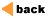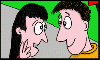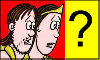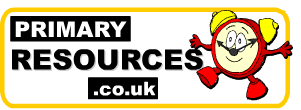Advertisement

Our Other Site: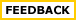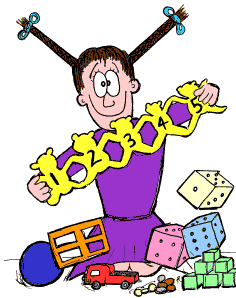Maths Words Make some cards, like the ones shown below, and get the children to group together ones that mean add, take away, times and share. They could stick the cards on a piece of paper divided up into four sections. It makes a useful wall reference chart. The children could even paste on some sample questions to show what type of calculations they are.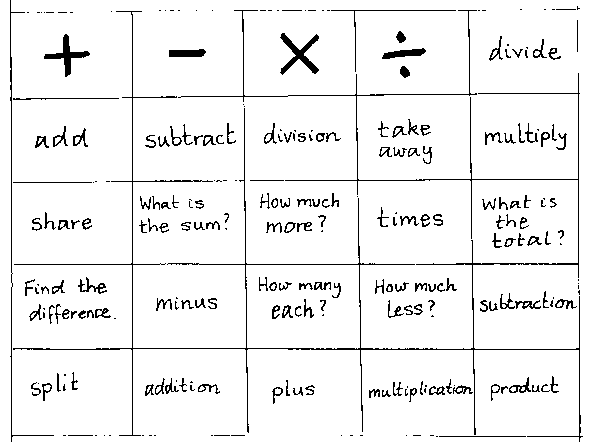Add, take away, share, times or a mixture?

 Joan has 12 sweeets. She has twice as many sweets as Leela. how many has Joan got? Find the total cost of 5 skirts and 5 shirts. If one shirt cost £3 and one skirt costs £4. 2 sets of 5 make? How many piles of 5 can you put 20 counters in? John and his two friends have 9 sweets. How many sweets do they have each? How many 26p stamps can you buy with £2.08? There are 16 children. How many tables will they need if each table sits 4 children? James has twice as many sweets as Bob. Bob has 5 sweets. How many sweets does James have? Ann had 32 points then she scored 7 more. How many points does she have altogether? 6 lots of 5 are? 5 piles of 4 give? Half of 6. Two threes. Two more than three. Find the sum of 1 and 9. The sum of two numbers is 9. One number is 6. What is the other? Increase 3 by 2. Decrease 5 by 2. Two less than five. Double 10.